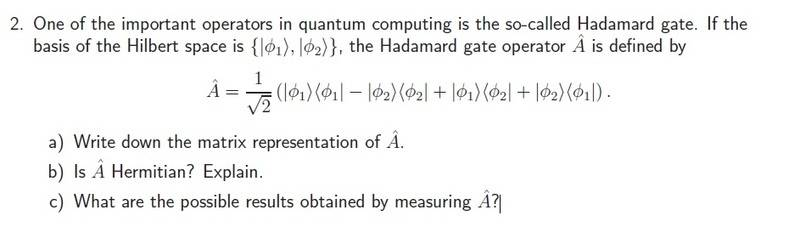# QM: "What are the possible results of measuring Operator A?"

## Homework Statement## The Attempt at a Solution

I'm fine with parts a) and b)

However I don't understand what part c) is asking me to do. How do I 'measure' an operator?

There are only two things I can think to do:
1. Find the expectation values of A for <Φ1|A|Φ1> and <Φ2|A|Φ2>
or
2. Find the operator eigenvalues of A

I know how to perform these tasks, so the only thing I'm confused about is the wording. I don't really understand what is meant when it talks about 'measuring' an operator.

Thanks.

blue_leaf77
Homework Helper
It asks you to find the eigenvalues of A. Measuring a quantity represented by an operator ##\hat{O}## in system specified by a state ##|\psi\rangle## mathematically translates into calculating ##\langle \psi|\hat{O}|\psi \rangle##. Now the system state ##|\psi\rangle## can be expanded into basis of ##\hat{O}##, performing this you will get ##\psi|\hat{O}|\psi \rangle=\sum_n |c_n|^2 O_n## where ##O_n## an eigenvalue of the operator and ##|c_n|^2 = |\langle o_n|\psi\rangle|^2## the probability of obtaining a value ##O_n## during the measurement.

•sa1988
It asks you to find the eigenvalues of A. Measuring a quantity represented by an operator ##\hat{O}## in system specified by a state ##|\psi\rangle## mathematically translates into calculating ##\langle \psi|\hat{O}|\psi \rangle##. Now the system state ##|\psi\rangle## can be expanded into basis of ##\hat{O}##, performing this you will get ##\psi|\hat{O}|\psi \rangle=\sum_n |c_n|^2 O_n## where ##O_n## an eigenvalue of the operator and ##|c_n|^2 = |\langle o_n|\psi\rangle|^2## the probability of obtaining a value ##O_n## during the measurement.

Thanks for that.

So essentially the eigenvalues of the operator are the only possible results from measuring the observable. Right?

I've seen examples applied to other problems where the we have energy eigenvalues that attribute to each possible state. I don't understand how the vectors come into it though? Are the vectors (kets) just ways of storing the information about the thing the eigenvalue is attributed to?

For example, a case where we have an energy value ε for some eigenvector |Ψ>, it's essentially saying that the 'stuff' within |Ψ> exists at an energy of ε ...?

blue_leaf77
Homework Helper
So essentially the eigenvalues of the operator are the only possible results from measuring the observable. Right?
Right.
I don't understand how the vectors come into it though? Are the vectors (kets) just ways of storing the information about the thing the eigenvalue is attributed to?
Yes, one can say that the state of a system is more or less the information carrying agent responsible for that system. The state dictates how the fluctuative behavior of measurement outcomes look like, i.e. whether or not there is uncertainty, and if there is, how of the possible outcomes are spread.
For example, a case where we have an energy value ε for some eigenvector |Ψ>, it's essentially saying that the 'stuff' within |Ψ> exists at an energy of ε ...?
If a system turns out to be in an eigenstate of the corresponding Hamiltonian, then a measurement of energy will always yield that same amount of energy which is the eigenvalue corresponding to the state the system is in. No matter how many times you run this measurement, you will always get the same result. Which means in this case there is no uncertainty.

•sa1988
Got it. Great stuff, thanks a lot.